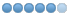# classicLadder doesnt work as i want

• thang
• Offline
• Elite Member
•27 Dec 2018 08:01 - 27 Dec 2018 08:19 #122942 by thang
Hi,

I'm using classicladder to connect modbus. there are some problems when i'm using it.

1st:When i use this config(didnt creat rungs yet):
loadrt classicladder_rt numRungs=150 numBits=20 numWords=2000 numTimers=10 numMonostables=10 numCounters=10 numSymbols=500 numPhysInputs=50 numPhysOutputs=50 numArithmExpr=500 numSections=4 numSymbols=500 numS32in=168 numS32out=100
If i increase numS32in > 168, then i cant set Bit Memory"%B". every plus 1, i lost 4 %B (like S32in = 169, then i cant set %B0-3)

2nd: When i start connecting 168 S32 Hal Pins (%IW) to Word memory(%W) by " ASSIGNMENT", i cant set "%Q" and "%B"
Last edit: 27 Dec 2018 08:19 by thang.

• cmorley
• Offline
• Moderator
•30 Dec 2018 05:47 #123131 by cmorley
when you say you cant assign b3 how are you assigning it?
I tried this with out modbus and with 168 s32, I could set b3.

Can you this confirm?

Chris M

• thang
• Offline
• Elite Member
•08 Jan 2019 07:36 - 08 Jan 2019 07:37 #123757 by thang
loadrt classicladder_rt numRungs=150 numBits=20 numWords=2000 numTimers=10 numMonostables=10 numCounters=10 numSymbols=500 numPhysInputs=50 numPhysOutputs=50 numArithmExpr=500 numSections=4 numSymbols=500 numS32in=168 numS32out=100 numFloatIn=1 numFloatOut=1

sorry, i miss last two parameters. When i increase numFloatIn and numFloatOut , It 's fixed
Last edit: 08 Jan 2019 07:37 by thang.